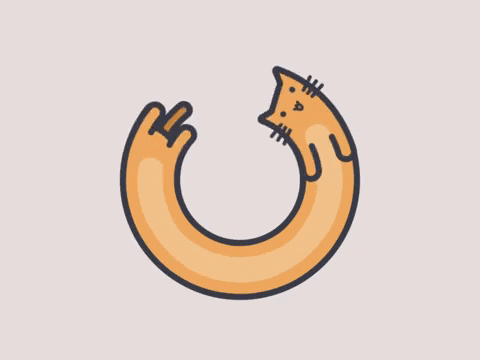## # 形状-刚体

### # 形状-刚体-关节

box2d 中创建刚体、关节的过程类似于堆积木，由形状构成刚体，由刚体构成关节，稍微不同的地方是，这里的形状可以多次使用，其过程： `形状 -> 刚体 -> 关节`

## # 形状

### # 形状性质

• 摩擦和恢复

• 密度

Box2D 可以根据附加形状的质量分配来计算物体的质量以及转动惯量。

### # 形状定义

• `b2ShapeDef`

• type 来表示形状类型
• userdata 表示用户数据
• localPosition 来表示当前位置
• localRotation 来表示当前角度
• friction、density、restitution 来表示摩擦力、密度、弹性系数
• groupIndex 来表示组号，这个组号可以用来过滤还比位标识优先
• `b2CircleDef`

• 继承于 b2ShapeDef
• type 为 e_circleShape
• `b2BoxDef`

• 继承于 b2ShapeDef
• type 为 e_ boxShape
• extents 来表示区域值
• `b2PolyDef`

• 继承于 b2ShapeDef
• type 为 e_ polyShape
• vertices 来表示顶点
• vertexCount 来表示顶点数，目前顶点数最多支持 8 个

## # 矩形

### # b2BoxDef

• `b2BoxDef`
• 继承于 b2ShapeDef
• type 为 e_ boxShape
• extents 来表示区域值
``````var Shape = new b2BoxDef(); //创建一个形状Shape，然后设置有关Shape的属性
Shape.extents.Set(1200, 5); //设置矩形高、宽,
Shape.density = 0; //设置矩形的密度
Shape.restitution = 0.3; //设置矩形的弹性
Shape.friction = 1; //设置矩形的摩擦因子，可以设置为0-1之间任意一个数，0表示光滑，1表示强摩擦
``````

## # 圆形

### # b2CircleDef

• `b2CircleDef`
• 继承于 b2ShapeDef
• type 为 e_circleShape
``````var Shape = new b2CircleDef(); //创建一个圆形Shape，然后设置有关Shape的属性
Shape.localPosition.Set(0, 0); //设置圆形的偏移量
Shape.density = 1.0; //设置圆形的密度
Shape.restitution = 0.3; //设置圆形的弹性
Shape.friction = 1; //设置圆形的摩擦因子
``````

## # 凸多边形

### # b2PolyDef

• `b2PolyDef`
• 继承于 b2ShapeDef
• type 为 e_ polyShape
• vertices 来表示顶点
• vertexCount 来表示顶点数，目前顶点数最多支持 8 个
``````var Shape = new b2PolyDef(); //创建一个多边形Shape，然后设置有关Shape的属性
Shape.vertexCount = 5; //设置多边形的顶点数，这里设置为5，意味着Shape是个五边形
Shape.vertices = new b2Vec2(0, -20); //分别定义五个顶点的坐标
Shape.vertices = new b2Vec2(25, 0);
Shape.vertices = new b2Vec2(15, 30);
Shape.vertices = new b2Vec2(-15, 30);
Shape.vertices = new b2Vec2(-25, 0);
Shape.localPosition.Set(0, 30); //设置多边形的偏移量
Shape.density = 1.0; //设置多边形的密度
Shape.restitution = 0.3; //设置多边形的弹性
Shape.friction = 1; //设置多边形的摩擦因子
``````

## # 刚体

### # 刚体定义(b2BodyDef)

• 质量性质

1. 在刚体定义中显式地设置
2. 显式地在刚体上设置(在其创建之后)
3. 基于刚体上形状之密度设置 在很多游戏环境中，根据形状密度计算质量是有意义的。这能帮助确保刚体有合理和一致的质量。然而，其它游戏环境可能需要指定质量值。例如，可能你有一个机械装置，需要一个精确的质量。
• 位置和角度

``````bodyDef.position.Set(x, y); // 设置刚体的初始位置
``````

• 阻尼

``````bodyDef.linearDamping = 0.0;
bodyDef.angularDamping = 0.01;
``````

• 休眠参数

``````bodyDef.allowSleep = true;
bodyDef.isSleeping = false;
``````

## # 由形状到刚体

### # b2BodyDef

``````var BodyDef = new b2BodyDef(); // 利用b2BodyDef类创建一个刚体
BodyDef.position.Set(0, 600); // 设置刚体的初始位置，这里把BodyDef放在（0，600）